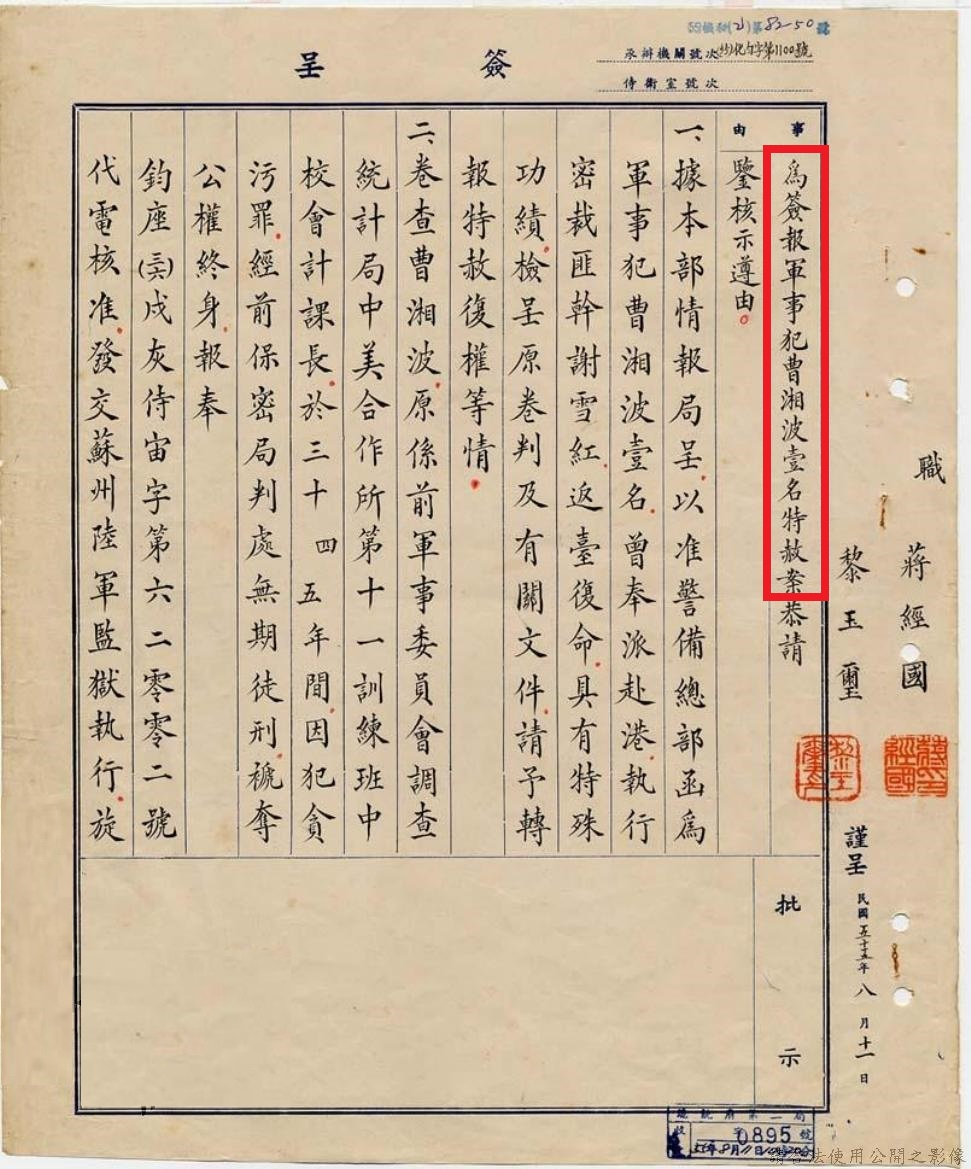# 【邱國禎】刺殺謝雪紅的大疑案「三十九年四月一日，前台灣省保安司令保安處副處長李葆初接獲香港密報，略謂『前台灣二二八事變禍首（共匪台灣民主自治同盟負責人）謝逆雪紅，近常往來港滬，負責策動台灣青年發展地下武裝』，經該處研判結果，以當時奸匪正積極準備攻台，謝匪在港之活動實為佈署攻台內應工作，對保衛本島構成重大威脅」。

「簽擬派員將該匪密予制裁，呈奉該部彭前副司令批示『奉諭照辦』，旋即擬具計畫，並派遣曹湘波及林元龍等五員，於同月十三日分乘船機赴港，幾經策畫佈署偵查跟蹤，直至六月十三日下午四時許，始在香港大酒店二樓，由曹員發槍三發，將謝匪擊倒，翌日返台復命，曾蒙頒發獎金新台幣五萬元在案。」【註一】

【註一】檔案管理局，檔號：B3750347701=0055=3136177=177=1=001=0005003420001

【註二】檔案管理局，檔號：AA01010000C=0039=301.1=0288=0001=virtual001=0051

【註三】檔案管理局，檔號：AA01010000C=0039=301.1=0288=0001=virtual001=0042

【註四】檔案管理局，檔號：AA01010000C=0039=301.1=0288=0001=virtual001=0040

【註五】檔案管理局，檔號：AA01010000C=0039=304.2=0518=0001=virtual001=0137

【註六】（五○）監臺院機字第七十三號通過彈劾，公務員懲戒委員會五十年九月二十二日台會議函字第八六四號議決降級處分。

【註七】（五五）監台院機字第○二四四號通過彈劾。

【註八】一九六五年九月二十日，台灣警備總司令部〈五四〉論寰字第六六三一號代電。檔案管理局檔號AA01010000C=0039=301.1=0288=0001=virtual001=0014。

【註九】檔案管理局，檔號：A305050000C=0036=0410.9=04601017=2=069=0004

【註十】引自陳芳明《謝雪紅評傳》的年表。

【註十一】同上。

【註十二】《台魂淚（三）：我們的遭遇》，作者：楊克煌、謝雪紅，台灣中央研究院台灣史研究所出版，p202。